# XGBoost in R Programming

XGBoost is a fast and efficient algorithm and used by winners of many machine learning competitions. XG Boost works only with the numeric variables. It is a part of the boosting technique in which the selection of the sample is done more intelligently to classify observations. There are interfaces of XGBoost in C++, R, Python, Julia, Java, and Scala. The core functions in XGBoost are implemented in C++, thus it is easy to share models among different interfaces. Based on the statistics from the CRAN mirror, the package has been downloaded for more than 81, 000 times. XgBoost modeling consists of two techniques: Bagging and Boosting.

• Bagging: It is an approach where you can take random samples of data, build learning algorithms and take simple means to find bagging probabilities.
• Boosting: It is an approach where selection of approach is made more intelligently i.e more an more weight is given to classify observations.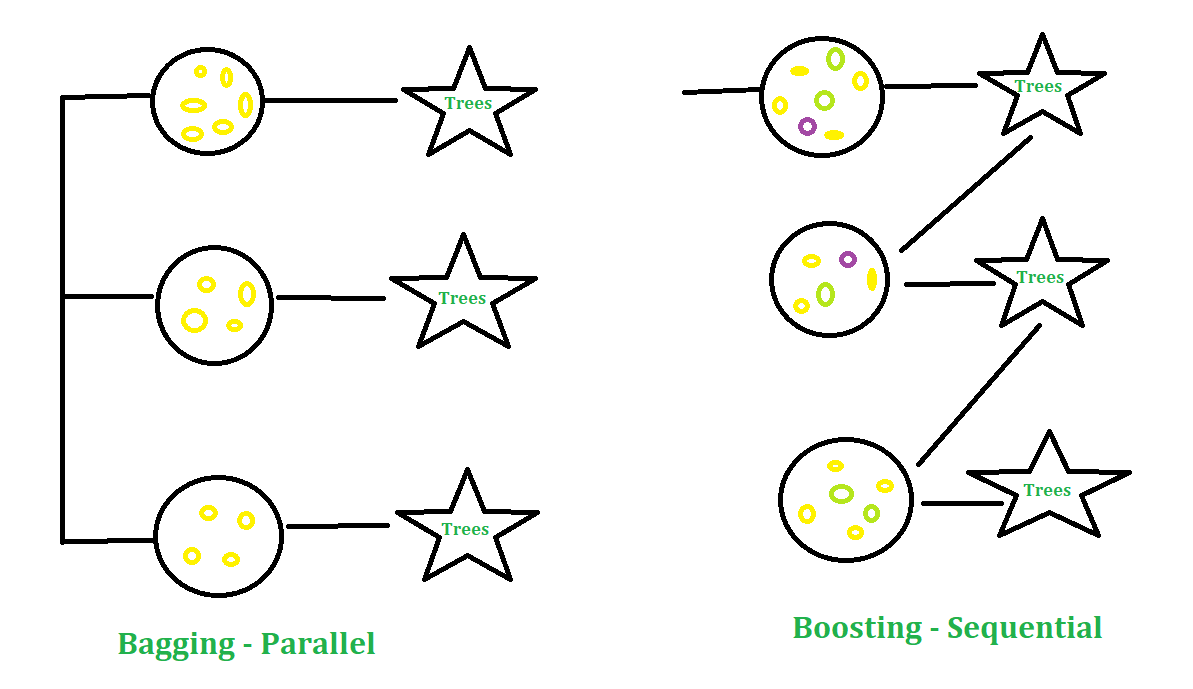#### Parameters in XGBoost

• eta: It shrinks the feature weights to make the boosting process more conservative. The range is from 0 to 1. It is also knowm as learning rate or Shrinking factor. Low eta value signifies the model is more robust to overfitting.
• gamma: The larger the value of gamma, more conservative the algorithm will be. It’s range is from 0 to infinity.
• max_depth: The maximum depth of a tree can be specified using `max_depth` parameter.
• Subsample: It is the proportion of rows that the model will randomly select to grow trees.
• colsample_bytree: It is the ratio of variables randomly chosen to build each tree in the model.

#### The Dataset

Big Mart dataset consists of 1559 products across 10 stores in different cities. Certain attributes of each product and store have been defined. It consists of 12 features i.e Item_Identifier( is a unique product ID assigned to every distinct item), Item_Weight(includes the weight of the product), Item_Fat_Content(describes whether the product is low fat or not), Item_Visibility(mentions the percentage of the total display area of all products in a store allocated to the particular product), Item_Type(describes the food category to which the item belongs), Item_MRP(Maximum Retail Price (list price) of the product), Outlet_Identifier(unique store ID assigned. It consists of an alphanumeric string of length 6), Outlet_Establishment_Year(mentions the year in which store was established), Outlet_Size(tells the size of the store in terms of ground area covered), Outlet_Location_Type(tells about the size of the city in which the store is located), Outlet_Type(tells whether the outlet is just a grocery store or some sort of supermarket) and Item_Outlet_Sales( sales of the product in the particular store).

 `# Loading data ` `train = ``fread``(``"Train_UWu5bXk.csv"``) ` `test = ``fread``(``"Test_u94Q5KV.csv"``) ` `  `  `# Structure  ` `str``(train) `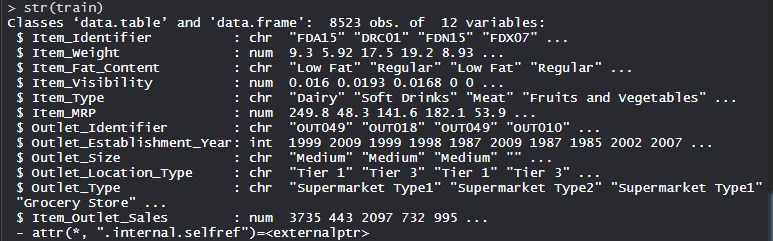#### Performing XGBoost on Dataset

Using XGBoost algorithm on the dataset which includes 12 features with 1559 products across 10 stores in different cities.

 `# Installing Packages ` `install.packages``(``"data.table"``) ` `install.packages``(``"dplyr"``) ` `install.packages``(``"ggplot2"``) ` `install.packages``(``"caret"``) ` `install.packages``(``"xgboost"``) ` `install.packages``(``"e1071"``) ` `install.packages``(``"cowplot"``) ` ` `  `# Loading packages ` `library``(data.table) ``# for reading and manipulation of data ` `library``(dplyr)      ``# for data manipulation and joining ` `library``(ggplot2)    ``# for ploting  ` `library``(caret)      ``# for modeling ` `library``(xgboost)    ``# for building XGBoost model ` `library``(e1071)      ``# for skewness ` `library``(cowplot)    ``# for combining multiple plots  ` ` `  `# Setting test dataset ` `# Combining datasets ` `# add Item_Outlet_Sales to test data ` `test[, Item_Outlet_Sales := ``NA``]  ` `combi = ``rbind``(train, test) ` ` `  `# Missing Value Treatment ` `missing_index = ``which``(``is.na``(combi\$Item_Weight)) ` `for``(i ``in` `missing_index){ ` `  ``item = combi\$Item_Identifier[i] ` `  ``combi\$Item_Weight[i] = ``mean``(combi\$Item_Weight ` `                         ``[combi\$Item_Identifier == item],  ` `                         ``na.rm = T) ` `} ` ` `  `# Replacing 0 in Item_Visibility with mean ` `zero_index = ``which``(combi\$Item_Visibility == 0) ` `for``(i ``in` `zero_index){ ` `  ``item = combi\$Item_Identifier[i] ` `  ``combi\$Item_Visibility[i] = ``mean``( ` `       ``combi\$Item_Visibility[combi\$Item_Identifier == item],  ` `       ``na.rm = T) ` `} ` ` `  `# Label Encoding ` `# To convert categorical in numerical ` `combi[, Outlet_Size_num :=  ` `           ``ifelse``(Outlet_Size == ``"Small"``, 0, ` `           ``ifelse``(Outlet_Size == ``"Medium"``, 1, 2))] ` ` `  `combi[, Outlet_Location_Type_num :=  ` `           ``ifelse``(Outlet_Location_Type == ``"Tier 3"``, 0, ` `           ``ifelse``(Outlet_Location_Type == ``"Tier 2"``, 1, 2))] ` ` `  `combi[, ``c``(``"Outlet_Size"``, ``"Outlet_Location_Type"``) := ``NULL``] ` ` `  `# One Hot Encoding ` `# To convert categorical in numerical ` `ohe_1 = ``dummyVars``(``"~."``, ` `        ``data = combi[, -``c``(``"Item_Identifier"``,  ` `                    ``"Outlet_Establishment_Year"``, ` `                    ``"Item_Type"``)], fullRank = T) ` `ohe_df = ``data.table``(``predict``(ohe_1,  ` `         ``combi[, -``c``(``"Item_Identifier"``,  ` `         ``"Outlet_Establishment_Year"``, ``"Item_Type"``)])) ` ` `  `combi = ``cbind``(combi[, ``"Item_Identifier"``], ohe_df) ` ` `  `# Remove skewness ` `skewness``(combi\$Item_Visibility)  ` `skewness``(combi\$price_per_unit_wt) ` ` `  `# log + 1 to avoid division by zero ` `combi[, Item_Visibility := ``log``(Item_Visibility + 1)]  ` ` `  `# Scaling and Centering data ` `# index of numeric features ` `num_vars = ``which``(``sapply``(combi, is.numeric))  ` `num_vars_names = ``names``(num_vars) ` ` `  `combi_numeric = combi[, ``setdiff``(num_vars_names,  ` `                ``"Item_Outlet_Sales"``), with = F] ` ` `  `prep_num = ``preProcess``(combi_numeric, ` `                 ``method = ``c``(``"center"``, ``"scale"``)) ` `combi_numeric_norm = ``predict``(prep_num, combi_numeric) ` ` `  `# removing numeric independent variables ` `combi[, ``setdiff``(num_vars_names,  ` `               ``"Item_Outlet_Sales"``) := ``NULL``]  ` `combi = ``cbind``(combi,  ` `              ``combi_numeric_norm) ` ` `  `# Splitting data back to train and test ` `train = combi[1:``nrow``(train)] ` `test = combi[(``nrow``(train) + 1):``nrow``(combi)] ` ` `  `# Removing Item_Outlet_Sales ` `test[, Item_Outlet_Sales := ``NULL``]  ` ` `  `# Model Building: XGBoost ` `param_list = ``list``( ` `  ``objective = ``"reg:linear"``, ` `  ``eta = 0.01, ` `  ``gamma = 1, ` `  ``max_depth = 6, ` `  ``subsample = 0.8, ` `  ``colsample_bytree = 0.5 ` `) ` ` `  `# Converting train and test into xgb.DMatrix format ` `Dtrain = ``xgb.DMatrix``( ` `         ``data = ``as.matrix``(train[, -``c``(``"Item_Identifier"``,  ` `                                ``"Item_Outlet_Sales"``)]),  ` `         ``label = train\$Item_Outlet_Sales) ` `Dtest = ``xgb.DMatrix``( ` `         ``data = ``as.matrix``(test[, -``c``(``"Item_Identifier"``)])) ` ` `  `# 5-fold cross-validation to  ` `# find optimal value of nrounds ` `set.seed``(112)  ``# Setting seed ` `xgbcv = ``xgb.cv``(params = param_list,  ` `               ``data = Dtrain,  ` `               ``nrounds = 1000,  ` `               ``nfold = 5,  ` `               ``print_every_n = 10,  ` `               ``early_stopping_rounds = 30,  ` `               ``maximize = F) ` ` `  `# Training XGBoost model at nrounds = 428 ` `xgb_model = ``xgb.train``(data = Dtrain,  ` `                      ``params = param_list,  ` `                      ``nrounds = 428) ` `xgb_model ` ` `  `# Variable Importance ` `var_imp = ``xgb.importance``( ` `             ``feature_names = ``setdiff``(``names``(train),  ` `             ``c``(``"Item_Identifier"``, ``"Item_Outlet_Sales"``)),  ` `             ``model = xgb_model) ` ` `  `# Importance plot ` `xgb.plot.importance``(var_imp) `

Output:

• Training of Xgboost model: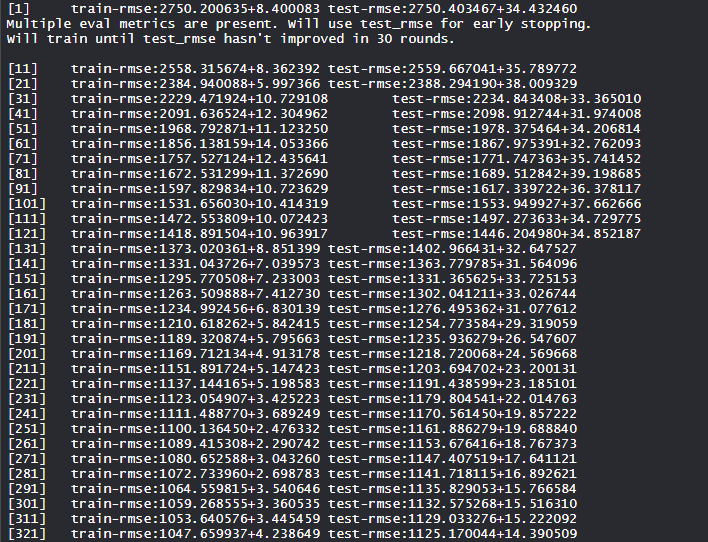The xgboost model is trained calculating the train-rmse score and test-rmse score and finding its lowest value in many rounds.

• Model xgb_model: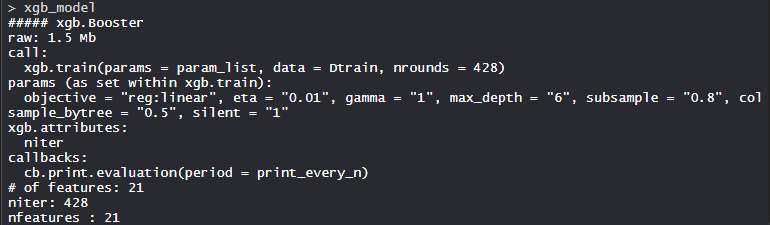The XgBoost models consist of 21 features with the objective of regression linear, eta is 0.01, gamma is 1, max_depth is 6, subsample is 0.8, colsample_bytree = 0.5 and silent is 1.

• Variable Importance plot: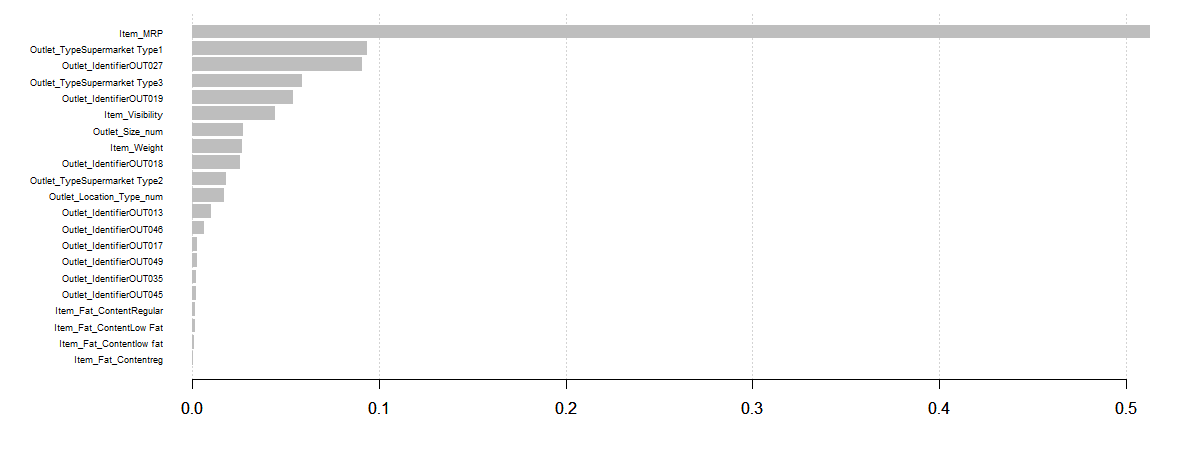The Item_MRP is the most important variable followed by Item_Visibility and Outlet_Location_Type_num.

So, Xgboost finds its applications in many sectors of industries and used with full capacity.

My Personal Notes arrow_drop_upCheck out this Author's contributed articles.

If you like GeeksforGeeks and would like to contribute, you can also write an article using contribute.geeksforgeeks.org or mail your article to contribute@geeksforgeeks.org. See your article appearing on the GeeksforGeeks main page and help other Geeks.

Please Improve this article if you find anything incorrect by clicking on the "Improve Article" button below.

Article Tags :

1

Please write to us at contribute@geeksforgeeks.org to report any issue with the above content.